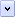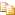In JScript, there are three primary data types, two composite data types, and two special data types.

# Primary Data Types

The primary (primitive) data types are:

• String

• Number

• Boolean

# Composite Data Types

The composite (reference) data types are:

• Object

• Array

# Special Data Types

The special data types are:

• Null

• Undefined

# String Data Type

A string value is a chain of zero or more Unicode characters (letters, digits, and punctuation marks) strung together. You use the string data type to represent text in JScript. String literals can be included in your scripts by enclosing them in matching pairs of single or double quotation marks. Double quotation marks can be contained within strings surrounded by single quotation marks, and single quotation marks can be contained within strings surrounded by double quotation marks. The following are examples of strings:Copy Code
```"Happy am I; from care I'm free!"
'"Avast, ye lubbers!" roared the technician.'
"42"
'c'```

Notice that JScript does not have a type to represent a single character. To represent a single character in JScript, you create a string that consists of only one character. A string that contains zero characters ("") is an empty (zero-length) string.

# Number Data Type

In JScript, there is no distinction between integer and floating-point values; a JScript number can be either (internally, JScript represent all numbers as floating-point values).

### Integer Values

Integer values can be positive whole numbers, negative whole numbers, and 0. They can be represented in base 10 (decimal), base 8 (octal), and base 16 (hexadecimal). Most numbers in JScript are written in decimal. You denote octal integers by prefixing them with a leading "0" (zero). They can contain digits 0 through 7 only. A number with a leading "0", containing the digits "8" and/or "9" is interpreted as a decimal number.

You denote hexadecimal ("hex") integers by prefixing them with a leading "0x" (zero and x|X). They can contain digits 0 through 9, and letters A through F (either uppercase or lowercase) only. The letters A through F are used to represent, as single digits, 10 through 15 in base 10. That is, 0xF is equivalent to 15, and 0x10 is equivalent to 16.

Both octal and hexadecimal numbers can be negative, but cannot have a decimal portion, and cannot be written in scientific (exponential) notation.

### Floating-point Values

Floating-point values can be whole numbers with a decimal portion. Additionally, they can be expressed in scientific notation. That is, an uppercase or lowercase "e" is used to represent "ten to the power of". JScript represents numbers using the eight byte IEEE 754 floating-point standard for numerical representation. This means you can write numbers as large as 1.7976931348623157x10308, and as small as 5x10-324. A number that begins with a single "0" and contains a decimal point is interpreted as a decimal floating-point number.

Notice that a number that begins with "0x" or "00" and contains a decimal point will generate an error. Here are some examples of JScript numbers.

Number Description Decimal Equivalent

.0001, 0.0001, 1e-4, 1.0e-4

Four equivalent floating-point numbers.

0.0001

3.45e2

A floating-point number.

345

42

An integer.

42

0378

An integer. Although this looks like an octal number (it begins with a zero), 8 is not a valid octal digit, so the number is treated as a decimal.

378

0377

An octal integer. Notice that although it only appears to be one less than the number above, its actual value is quite different.

255

0.0001

A floating point number. Even though this begins with a zero, it is not an octal number because it has a decimal point.

0.0001

00.0001

This is an error. The two leading zeros mark the number as an octal, but octals are not allowed a decimal component.

N/A (compiler error)

0Xff

255

0x37CF

14287

0x3e7

A hexadecimal integer. Notice that the 'e' is not treated as exponentiation.

999

0x3.45e2

This is an error. Hexadecimal numbers cannot have decimal parts.

N/A (compiler error)

Additionally, JScript contains numbers with special values. These are:

• NaN (not a number). This is used when a mathematical operation is performed on inappropriate data, such as strings or the undefined value

• Positive Infinity. This is used when a positive number is too large to represent in JScript

• Negative Infinity. This is used when a negative number is too large to represent in JScript

• Positive and Negative 0. JScript differentiates between positive and negative zero.

# Boolean Data Type

Whereas the string and number data types can have a virtually unlimited number of different values, the Boolean data type can only have two. They are the literals true and false. A Boolean value is a truth-value — it expresses the validity of a condition (tells whether the condition is true or not).

Comparisons you make in your scripts always have a Boolean outcome. Consider the following line of JScript code.Copy Code
`y = (x == 2000);`

Here, the value of the variable x is tested to see if it is equal to the number 2000. If it is, the result of the comparison is the Boolean value true, which is assigned to the variable y. If x is not equal to 2000, then the result of the comparison is the Boolean value false.

Boolean values are especially useful in control structures. Here, you combine a comparison that creates a Boolean value directly with a statement that uses it. Consider the following JScript code sample.Copy Code
```if (x == 2000)
z = z + 1;
else
x = x + 1;```

The if/else statement in JScript performs one action if a Boolean value is true (in this case, z = z + 1), and an alternate action if the Boolean value is false (x = x + 1).

You can use any expression as a comparative expression. Any expression that evaluates to 0, null, undefined, or an empty string is interpreted as false. An expression that evaluates to any other value is interpreted as true. For example, you could use an expression such as:Copy Code
`if (x = y + z) // This may not do what you expect — see below!`

Note that the above line does not check if x is equal to y + z, since only a single equal sign (assignment) is used. Instead, the code above assigns the value of y + z to the variable x, and then checks if the result of the entire expression (the value of x) is zero. To check if x is equal to y + z, use the following code.Copy Code
`if (x == y + z) // This is different to the code above!`

# Null Data Type

The null data type has only one value in JScript: null. The null keyword cannot be used as the name of a function or variable.

A variable that contains null contains "no value" or "no object." In other words, it holds no valid number, string, Boolean, array, or object. You can erase the contents of a variable (without deleting the variable) by assigning it the null value.

Notice that in JScript, null is not the same as 0 (as it is in C and C++). Also note that the typeof operator in JScript will report null values as being of type Object, not of type null. This potentially confusing behavior is for backwards compatibility.

# Undefined Data Type

The undefined value is returned when you use:

• an object property that does not exist,

• a variable that has been declared, but has never had a value assigned to it.

Notice that you cannot test to see if a variable exists by comparing it to undefined, although you can check if its type is "undefined". In the following code example, assume that the programmer is trying to test if the variable x has been declared:Copy Code
```// This method will not work
if (x == undefined)
// do something

// This method also won't work - you must check for
// the string "undefined"
if (typeof(x) == undefined)
// do something

// This method will work
if (typeof(x) == "undefined")
// do something```

Consider comparing the undefined value to null.Copy Code
`someObject.prop == null;`

This comparison is true,

• if the property someObject.prop contains the value null,

• if the property someObject.prop does not exist.

To check if an object property exists, you can use the new in operator:Copy Code
```if ("prop" in someObject)
// someObject has the property 'prop'```

Home